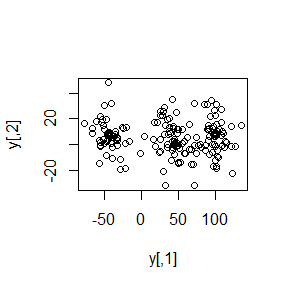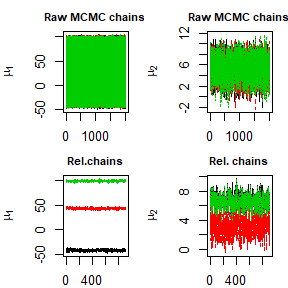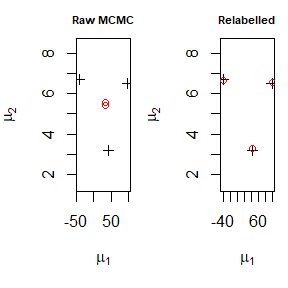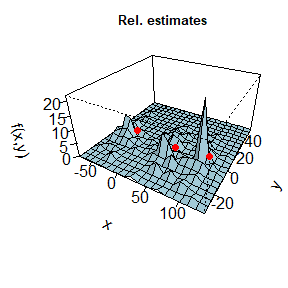# Dealing with label switching: relabelling in Bayesian mixture models by pivotal units

#### 2019-02-26

In this vignette we explore the relabelling pivotal method proposed in Egidi et al. (2018b) through the pivmet package. First of all, we load the package:

library(pivmet)

There are two main functions designed for our procedure. The function piv_MCMC:

• performs MCMC sampling for Gaussian mixture models launching the JAGSrun function of the bayesmix package for univariate models or the run.jags function of the runjags package for bivariate models;

• post-processes the chains and randomly swithes their values;

• builds a co-association matrix $$C$$ for the $$N$$ statistical units, where the single cell $$c_{ip}$$ is the fraction of times the unit $$i$$ and the unit $$p$$ belong to the same group along the $$H$$ MCMC iterations;

• extracts some pivotal units via the internal function piv_sel, one for each of the pre-specified $$k$$ groups.

The function piv_rel:

• performs the relabelling algorithm using the $$k$$ pivotal units as groups identifiers. It yields the relabelled chains and the corresponding posterior estimates.

## Example: bivariate data

Suppose $$\boldsymbol{y}_i \sim \sum_{j=1}^{k}\pi_{k}\mathcal{N}_{2}(\boldsymbol{\mu}_{k}, \boldsymbol{\Sigma})$$. We may generate Gaussian mixture data through the function piv_sim, specifying the desired number of groups $$k$$. The argument W handles the weights for a nested mixture, in which each $$j$$-th component is in turn modelled as a two-component mixture.

set.seed(50)
N  <- 200
k  <- 3
nMC <- 2000
M1 <- c(-45,8)
M2 <- c(45,.1)
M3 <- c(100,8)
Mu <- matrix(rbind(M1,M2,M3),c(k,2))
stdev    <- cbind(rep(1,k), rep(200,k))
Sigma.p1 <- matrix(c(stdev[1,1],0,0,stdev[1,1]),
nrow=2, ncol=2)
Sigma.p2 <- matrix(c(stdev[1,2],0,0,stdev[1,2]),
nrow=2, ncol=2)
W   <- c(0.2,0.8)
sim <- piv_sim(N = N, k = k, Mu = Mu,
stdev = stdev, Sigma.p1 = Sigma.p1, Sigma.p2 = Sigma.p2, W = W)
plot(sim$y, xlab="y[,1]", ylab="y[,2]")The function piv_MCMC requires only three mandatory arguments: data y, number of component k and number of MCMC iterations nMC. It performs JAGS sampling using the bayesmix package for univariate data and runjags package for bivariate data. After $$H$$ MCMC iterations, the function implements a clustering procedure on $$k$$ groups, and through the optional argument clustering the user may choose among agglomerative or divisive hierarchical clustering. Using the latent formulation for mixture models, we denote with $$[Z_i]_h$$ the group allocation of the $$i$$-th unit at the $$h$$-th iteration. The function builds a co-association matrix $$C$$ across the MCMC sample with generic element: $c_{ip} = \frac{n_{ip}}{H}=\frac{1}{H} \sum_{h=1}^{H}|[Z_i]_h=[Z_p]_h|,$ where $$|\cdot|$$ denotes the event indicator and $$n_{ip}$$ is the number of times the units $$i, \ p$$ belong to the same group along the sampling. Using this matrix, we may extract some units, one for each group, which are (pairwise) separated with (posterior) probability one (that is, the posterior probability of any two of them being in the same group is zero). We call them pivots, and denote them with: $$i_1,\ldots,i_k$$. With the optional argument piv.criterion, the user may choose among three (plus one) procedures for extracting the pivotal units. For group $$j$$ containing $$J_j$$ units, one can choose: • $$i^{*}$$ that maximizes $$\sum_{p \in J_j}c_{ip}$$ (maxsumint); • $$i^{*}$$ that maximizes $$\sum_{p \in J_j}c_{ip}- \sum_{p \not\in J_j}c_{ip}$$ (maxsumdiff, default method); • $$i^{*}$$ that minimizes $$\sum_{p \not\in J_j}c_{ip}$$ (minsumnoint). Alternatively, when $$k <5$$, the user has the optional pivotal criterion MUS (Egidi et al. 2018a) based on a sequential search within the matrix $$C$$. res <- piv_MCMC(y = sim$y, k= k, nMC =nMC)
#> Compiling rjags model...
#> Calling the simulation using the rjags method...
#> Note: the model did not require adaptation
#> Burning in the model for 1000 iterations...
#> Running the model for 2000 iterations...
#> Simulation complete
#> Note: Summary statistics were not produced as there are >50
#> monitored variables
#> [To override this behaviour see ?add.summary and ?runjags.options]
#> FALSEFinished running the simulation

Once we obtain posterior estimates, label switching is likely to occurr. For such a reason, we need to relabel our chains, and the pivotal units previously detected play a central role, yielding to the following relabelling:

\begin{align*} [\mu_{j}]_h=&[\mu_{Z_{i_{j}}}]_h \\ [Z_{i}]_h =j & \mbox{ for } i : [Z_i]_h=[Z_{i_{j}}]_h.\\ \end{align*}

The function piv_rel performs the procedure above, and for such task it only needs the mcmc = rel argument and nMC. Once we correctly relabel the chains, we may plot the relabelled outputs through the function piv_plot, with different options for the argument type:

• chains: plot the relabelled chains;
• estimates: plot the point estimates for the parameters of interest;
• estimates_hist: plot the point estimates against the histogram of the data;
• iter: plot the proportions of valid MCMC iterations.
rel <- piv_rel(mcmc=res, nMC = nMC)
piv_plot(y = y, mcmc = res, rel_est = rel, type = "chains")
piv_plot(y = y, mcmc = res, rel_est = rel, type = "estimates")
piv_plot(y = sim\$y, mcmc = res, rel_est = rel, type = "hist")